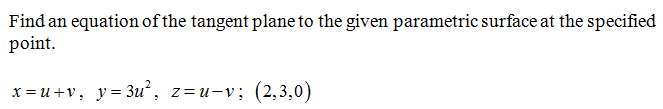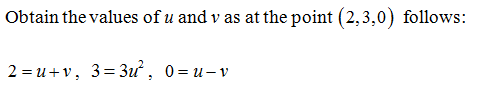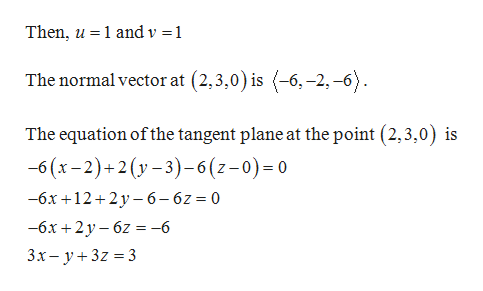# Find an equation of the tangent plane to the given parametric surface at the specified point. x = u + v,  y = 3u^2,  z = u - v;  (2,3,0)

Question
8 views

Find an equation of the tangent plane to the given parametric surface at the specified point.

x = u + v,  y = 3u^2,  z = u - v;  (2,3,0)

check_circle

Step 1Step 2...help_outlineImage TranscriptioncloseThen, u 1 and v = 1 The normal vector at (2,3,0) is (-6, -2,-6) The equation of the tangent plane at the point (2,3,0) is -6 (x-2)+2(y-3)-6 (z-0) 0 —6х +12+2у-6— 62 %3D 0 -6х +2 у-62 %3D -6 3х— у+3z %3D 3 fullscreen

### Want to see the full answer?

See Solution

#### Want to see this answer and more?

Solutions are written by subject experts who are available 24/7. Questions are typically answered within 1 hour.*

See Solution
*Response times may vary by subject and question.
Tagged in

### Calculus